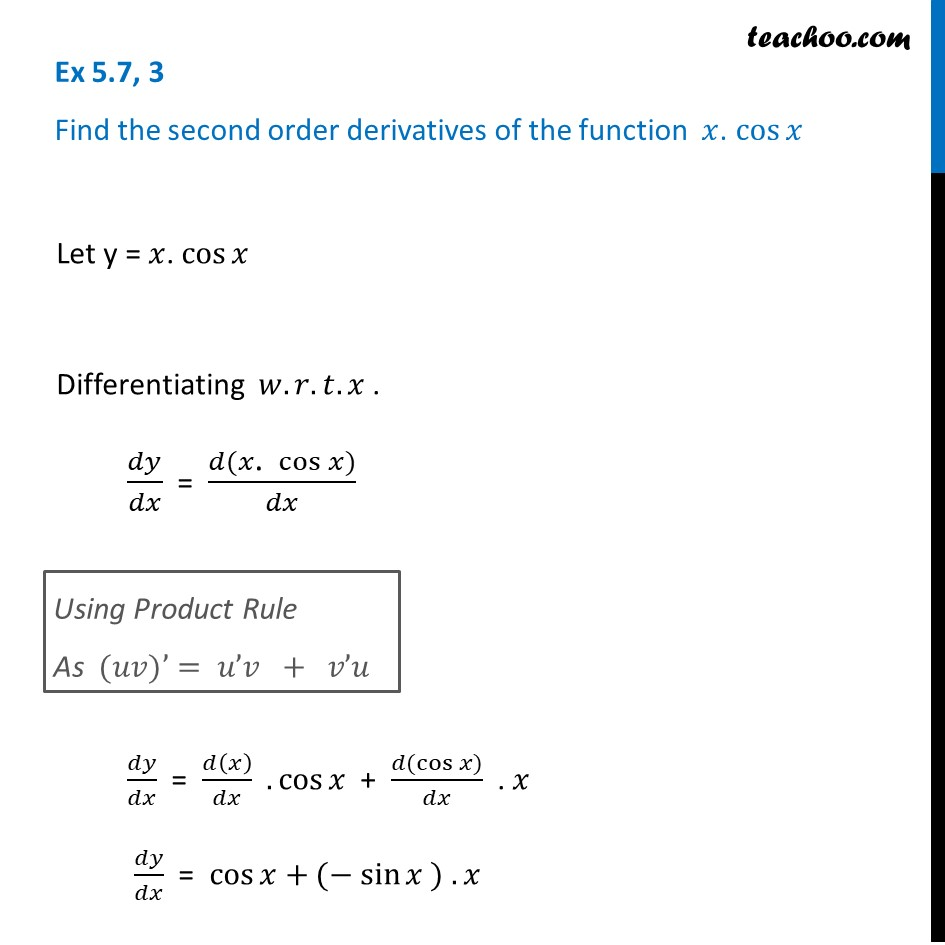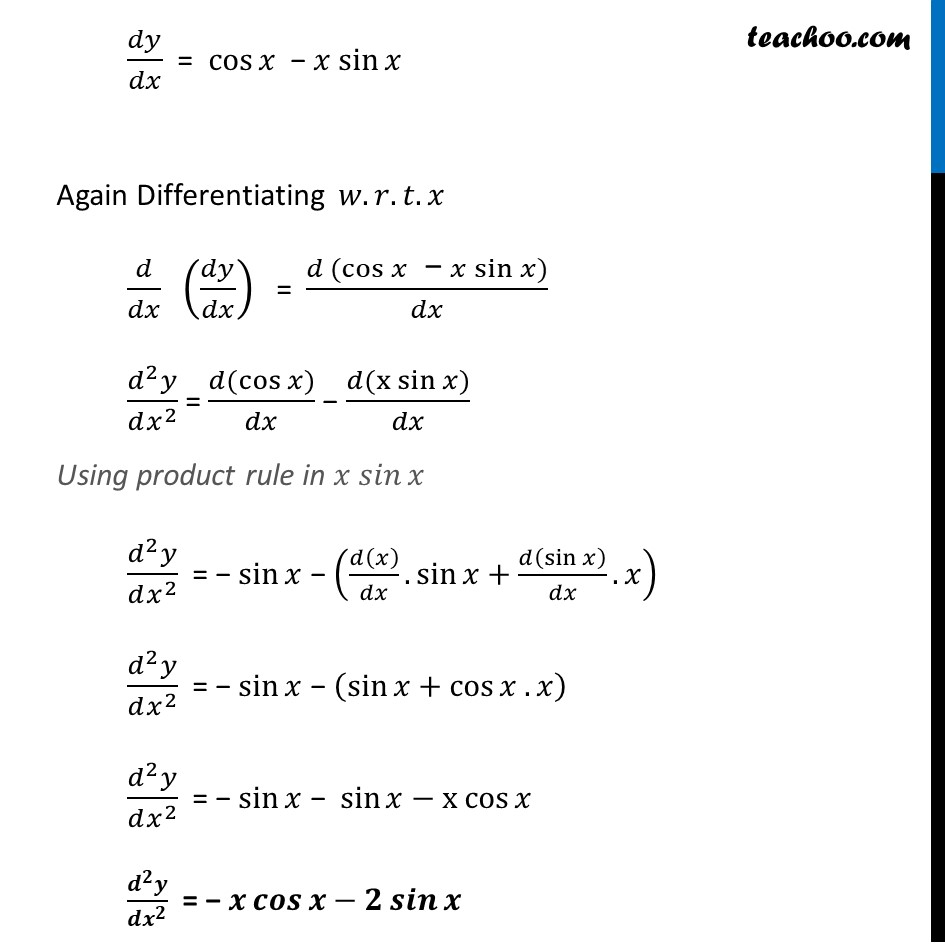Ex 5.7

Chapter 5 Class 12 Continuity and Differentiability
Serial order wiseLearn in your speed, with individual attention - Teachoo Maths 1-on-1 Class

### Transcript

Ex 5.7, 3 Find the second order derivatives of the function 𝑥. cos⁡𝑥 Let y = 𝑥. cos⁡𝑥 Differentiating 𝑤.𝑟.𝑡.𝑥 . 𝑑𝑦/𝑑𝑥 = (𝑑(𝑥". " cos⁡𝑥))/𝑑𝑥 𝑑𝑦/𝑑𝑥 = 𝑑(𝑥)/𝑑𝑥 .cos⁡𝑥 + (𝑑(cos⁡〖𝑥)〗)/𝑑𝑥 . 𝑥 𝑑𝑦/𝑑𝑥 = cos⁡𝑥+(− sin⁡𝑥 ) . 𝑥 Using Product Rule As (𝑢𝑣)’= 𝑢’𝑣 + 𝑣’𝑢 𝑑𝑦/𝑑𝑥 = cos⁡𝑥 − 𝑥 sin⁡𝑥 Again Differentiating 𝑤.𝑟.𝑡.𝑥 𝑑/𝑑𝑥 (𝑑𝑦/𝑑𝑥) = (𝑑 (cos⁡𝑥" − " 𝑥 sin⁡𝑥))/𝑑𝑥 (𝑑^2 𝑦)/(𝑑𝑥^2 ) = (𝑑(cos⁡〖𝑥)〗)/𝑑𝑥 − (𝑑(〖x sin〗⁡〖𝑥)〗)/𝑑𝑥 Using product rule in 𝑥 𝑠𝑖𝑛⁡𝑥" " (𝑑^2 𝑦)/(𝑑𝑥^2 ) = "− " sin⁡𝑥 − (𝑑(𝑥)/𝑑𝑥.sin⁡𝑥+𝑑(sin⁡𝑥 )/𝑑𝑥.𝑥) (𝑑^2 𝑦)/(𝑑𝑥^2 ) = "− " sin⁡𝑥 − (sin⁡〖𝑥+cos⁡〖𝑥 . 𝑥〗 〗 ) (𝑑^2 𝑦)/(𝑑𝑥^2 ) = "− " sin⁡𝑥 − sin⁡〖𝑥−〖x cos〗⁡〖𝑥 〗 〗 (𝒅^𝟐 𝒚)/(𝒅𝒙^𝟐 ) = "− " 𝒙 𝒄𝒐𝒔⁡𝒙−𝟐 𝒔𝒊𝒏⁡𝒙